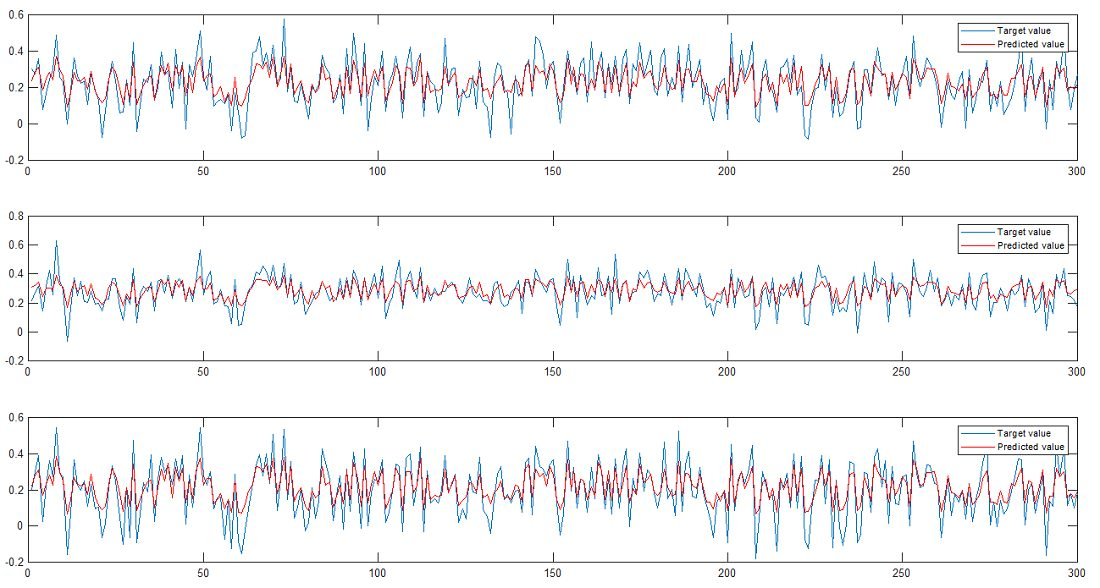Sale!

# MATLAB code for Gaussian Process-Based Regression Techniques

19

## Description

Gaussian-based regression is a strong, non-parametric Bayesian approach for solving the problems of regression techniques.

Gaussian Process has become a good method for non-parametric modeling, especially for time series data, and for various modeling tasks.MATLAB code for Gaussian Process-Based Regression Techniques

We did not use any MATLAB toolbox for the implementation of this code, we just use MATLAB basic programming.

## Reviews

There are no reviews yet.

SKU: 8RVMBYS95 Category: